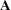WEIGHT Statement

WEIGHT variable ;

If you want to specify relative weights for each observation in the input data set, place the weights in a variable in the data set and specify that variable name in a WEIGHT statement. This is often done when the variance associated with each observation is different and the values of the weight variable are proportional to the reciprocals of the variances. The values of the WEIGHT variable can be nonintegral and are not truncated. An observation is used in the analysis only if the value of the WEIGHT variable is greater than zero.

The WEIGHT and FREQ statements have a similar effect, except in calculating the divisor of thematrix.